Technical Test SSC JE: Electrical Engineering (EE)- 9

# Technical Test SSC JE: Electrical Engineering (EE)- 9

Test Description

## 100 Questions MCQ Test Mock Test Series for SSC JE Electrical Engineering | Technical Test SSC JE: Electrical Engineering (EE)- 9

Technical Test SSC JE: Electrical Engineering (EE)- 9 for Electrical Engineering (EE) 2022 is part of Mock Test Series for SSC JE Electrical Engineering preparation. The Technical Test SSC JE: Electrical Engineering (EE)- 9 questions and answers have been prepared according to the Electrical Engineering (EE) exam syllabus.The Technical Test SSC JE: Electrical Engineering (EE)- 9 MCQs are made for Electrical Engineering (EE) 2022 Exam. Find important definitions, questions, notes, meanings, examples, exercises, MCQs and online tests for Technical Test SSC JE: Electrical Engineering (EE)- 9 below.
Solutions of Technical Test SSC JE: Electrical Engineering (EE)- 9 questions in English are available as part of our Mock Test Series for SSC JE Electrical Engineering for Electrical Engineering (EE) & Technical Test SSC JE: Electrical Engineering (EE)- 9 solutions in Hindi for Mock Test Series for SSC JE Electrical Engineering course. Download more important topics, notes, lectures and mock test series for Electrical Engineering (EE) Exam by signing up for free. Attempt Technical Test SSC JE: Electrical Engineering (EE)- 9 | 100 questions in 100 minutes | Mock test for Electrical Engineering (EE) preparation | Free important questions MCQ to study Mock Test Series for SSC JE Electrical Engineering for Electrical Engineering (EE) Exam | Download free PDF with solutions
 1 Crore+ students have signed up on EduRev. Have you?
Technical Test SSC JE: Electrical Engineering (EE)- 9 - Question 1

### For a single phase capacitor start induction motor which of the following is valid ?

Detailed Solution for Technical Test SSC JE: Electrical Engineering (EE)- 9 - Question 1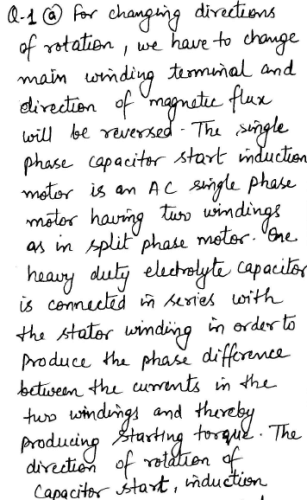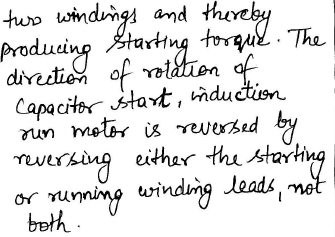Technical Test SSC JE: Electrical Engineering (EE)- 9 - Question 2

### To avoid arcing, connect suitable value of in earthing

Technical Test SSC JE: Electrical Engineering (EE)- 9 - Question 3

### What is the value of the current I in the circuit shown below ?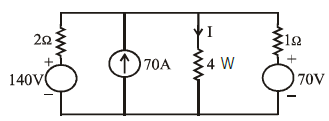Detailed Solution for Technical Test SSC JE: Electrical Engineering (EE)- 9 - Question 3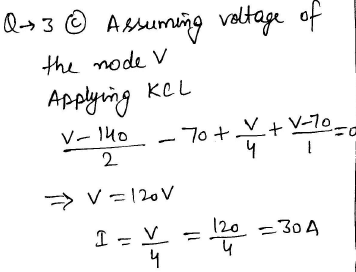Technical Test SSC JE: Electrical Engineering (EE)- 9 - Question 4

In the following type of conductors the corona is minimum in

Technical Test SSC JE: Electrical Engineering (EE)- 9 - Question 5

Consider the following w.r.t. the circuit as shown below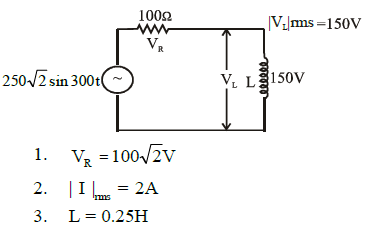Which of the above statements is/are correct?

Detailed Solution for Technical Test SSC JE: Electrical Engineering (EE)- 9 - Question 5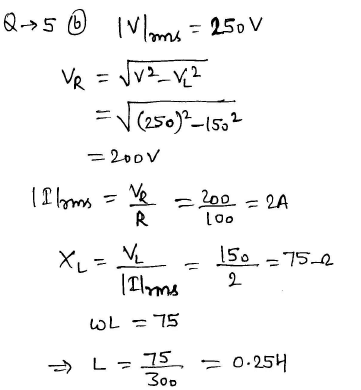Technical Test SSC JE: Electrical Engineering (EE)- 9 - Question 6

If the time delay of relay with TMS setting 0.1 is 10 second then what is the time delay when the TMS setting is changed to 0.5

Detailed Solution for Technical Test SSC JE: Electrical Engineering (EE)- 9 - Question 6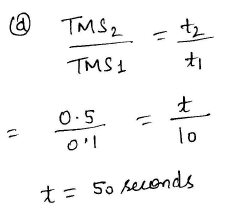Technical Test SSC JE: Electrical Engineering (EE)- 9 - Question 7

The machine used in wind will is

Technical Test SSC JE: Electrical Engineering (EE)- 9 - Question 8

In an RLC series resonant circuit, if the maximum stored energy is increased by 10%and at the same time, the energy dissipated per cycle is reduced by 10%, it will result in which one of the following ?

Detailed Solution for Technical Test SSC JE: Electrical Engineering (EE)- 9 - Question 8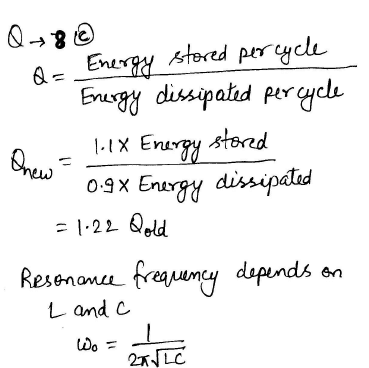Technical Test SSC JE: Electrical Engineering (EE)- 9 - Question 9

Consider the following components Out of these components, which component(s)can be common for primary & back up protection

Technical Test SSC JE: Electrical Engineering (EE)- 9 - Question 10

In a series resonance circuit, at resonance selectivity Q is equal to

Technical Test SSC JE: Electrical Engineering (EE)- 9 - Question 11

Positive sequence terminal voltage is zero in

Technical Test SSC JE: Electrical Engineering (EE)- 9 - Question 12

Most economical load in the underground cable is

Technical Test SSC JE: Electrical Engineering (EE)- 9 - Question 13

In distribution lines

Technical Test SSC JE: Electrical Engineering (EE)- 9 - Question 14

A certain network consist of a large number of ideal linear resistances, one of which is
designated consumed by R is P1 when only the first source is active, and P2 when only the
second source is active, if both source are active simultaneously, then the power consumed by R
is

Detailed Solution for Technical Test SSC JE: Electrical Engineering (EE)- 9 - Question 14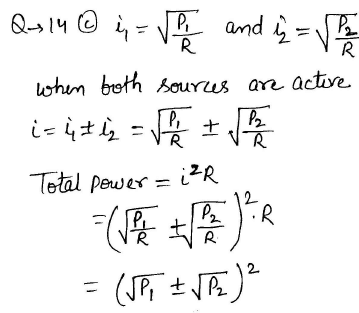Technical Test SSC JE: Electrical Engineering (EE)- 9 - Question 15

Consider the following statement about D'Arsonval Movement
1. It is best suited for d.c. current measurement
2. It responds to the average value of current
3. It could be used for power measurements

Which of these statements is/are correct

Detailed Solution for Technical Test SSC JE: Electrical Engineering (EE)- 9 - Question 15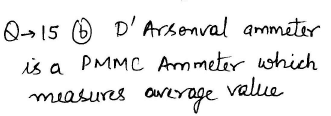Technical Test SSC JE: Electrical Engineering (EE)- 9 - Question 16

Torque/weight ratio of an instrument indicates

Detailed Solution for Technical Test SSC JE: Electrical Engineering (EE)- 9 - Question 16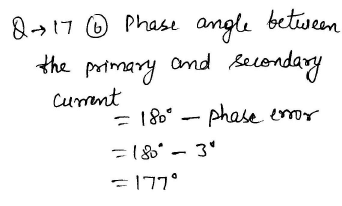Technical Test SSC JE: Electrical Engineering (EE)- 9 - Question 17

A current transformer has a phase error of +3º. The phase angle between the primary andsecondary current is

Detailed Solution for Technical Test SSC JE: Electrical Engineering (EE)- 9 - Question 17Technical Test SSC JE: Electrical Engineering (EE)- 9 - Question 18

The direction of rotation of a d.c. series motor can be changed by

Detailed Solution for Technical Test SSC JE: Electrical Engineering (EE)- 9 - Question 18

From speed current characteristics for a DC series motor, we get that speed is inversely proportional to flux. If flux direction is reversed, same flux will be available with negative sign in vectors, thus, speed will remain same but with the other direction.

Technical Test SSC JE: Electrical Engineering (EE)- 9 - Question 19

A three phase transformer having zero sequence impedance of Zo has the zero-sequence network as shown in the figure below. The connection of its windings are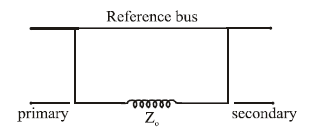Detailed Solution for Technical Test SSC JE: Electrical Engineering (EE)- 9 - Question 19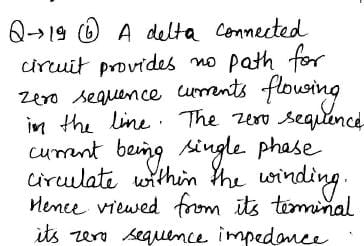Technical Test SSC JE: Electrical Engineering (EE)- 9 - Question 20

The transformer laminations are insulated from each other by

Technical Test SSC JE: Electrical Engineering (EE)- 9 - Question 21

A coil with certain number of turns has a specified time constant. If the no. of turns is doubled, its time constant would

Detailed Solution for Technical Test SSC JE: Electrical Engineering (EE)- 9 - Question 21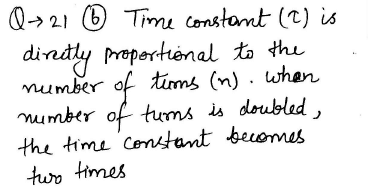Technical Test SSC JE: Electrical Engineering (EE)- 9 - Question 22

The technique of adding a precise amount of time between the trigger point and the beginning of the scope-sweep in a CRO is known as

Technical Test SSC JE: Electrical Engineering (EE)- 9 - Question 23

In a CE (common emitter) transistor, VCC = 12V and the zero signal collector current is 1mA. Determine the operating point when collector load (RC) is 6 KW

Technical Test SSC JE: Electrical Engineering (EE)- 9 - Question 24

A 100 H.P. motor with a synchronous speed of 720 rpm at 50 Hz has 96 stator slots with4 conductors per slot and 120 rotor slots with 2 conductors per slot. If the full load efficiency is 0.92 and the p.f. is 0.9 lagging, the current per phase when the stator is star connected to3-phase, 500V supply will be

Detailed Solution for Technical Test SSC JE: Electrical Engineering (EE)- 9 - Question 24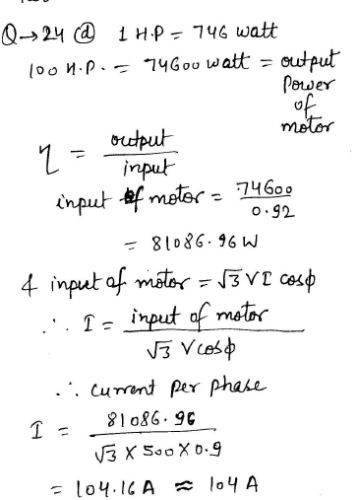Technical Test SSC JE: Electrical Engineering (EE)- 9 - Question 25

Which of the following application requires high starting torque ?

Technical Test SSC JE: Electrical Engineering (EE)- 9 - Question 26

Which DC motor will be preferred for machine tools ?

Technical Test SSC JE: Electrical Engineering (EE)- 9 - Question 27

The empty space between the plates of a capacitor is filled with liquid of dielectricconstant K the capacitance of capacitor

Detailed Solution for Technical Test SSC JE: Electrical Engineering (EE)- 9 - Question 27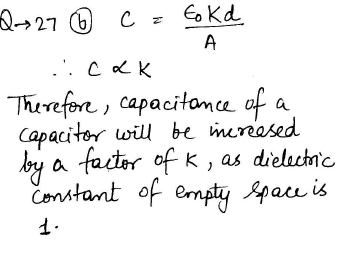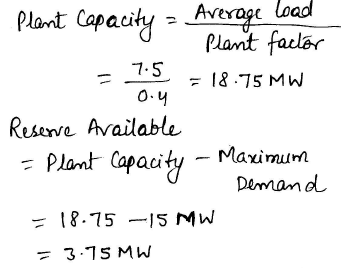Technical Test SSC JE: Electrical Engineering (EE)- 9 - Question 28

If current through the operating coil of a moving iron instrument is doubled, the operating forcebecomes

Technical Test SSC JE: Electrical Engineering (EE)- 9 - Question 29

A magnetic needle is kept in a uniform magnetic field. It experiences

Technical Test SSC JE: Electrical Engineering (EE)- 9 - Question 30

Technical Test SSC JE: Electrical Engineering (EE)- 9 - Question 31

For improvement of power factor

Technical Test SSC JE: Electrical Engineering (EE)- 9 - Question 32

A 4-pole 500V shunt motor has 720 wave connected conductors on its armature. The fullload armature current is 60A and flux per pole is 0.03 Wb. The armature resistance is 0.2 Wand the contact drop per brush is 1V. What is the back emf ?

Detailed Solution for Technical Test SSC JE: Electrical Engineering (EE)- 9 - Question 32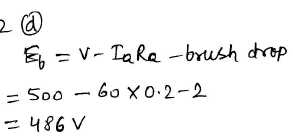Technical Test SSC JE: Electrical Engineering (EE)- 9 - Question 33

In an autotransformer of voltage ratio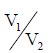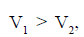the fraction of power transferred
inductively is proportional to

Technical Test SSC JE: Electrical Engineering (EE)- 9 - Question 34

Stepped core is used in transformers in order to reduce

Technical Test SSC JE: Electrical Engineering (EE)- 9 - Question 35

A make break switch is provided to disconnect the battery when the meter is not in use is

Technical Test SSC JE: Electrical Engineering (EE)- 9 - Question 36

Which instrument is the cheapest, disregarding the accuracy

Technical Test SSC JE: Electrical Engineering (EE)- 9 - Question 37

In dc machines, the armature reaction mmf is

Technical Test SSC JE: Electrical Engineering (EE)- 9 - Question 38

Inductance affects the flow of direct current at the time of

Detailed Solution for Technical Test SSC JE: Electrical Engineering (EE)- 9 - Question 38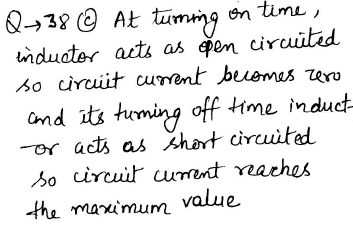Technical Test SSC JE: Electrical Engineering (EE)- 9 - Question 39

A centre zero ohmmeter connected in the rotor circuit of 6-pole 50 Hz induction motor makes30 oscillations in one minutes. The rotor speed is

Detailed Solution for Technical Test SSC JE: Electrical Engineering (EE)- 9 - Question 39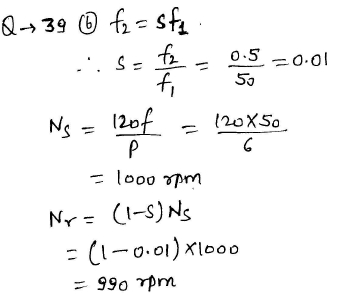Technical Test SSC JE: Electrical Engineering (EE)- 9 - Question 40

A coil of resistance 20W and inductance 0.8 H is connected to a 200V DC supply. The rate
of change of current at t = 0+ is

Detailed Solution for Technical Test SSC JE: Electrical Engineering (EE)- 9 - Question 40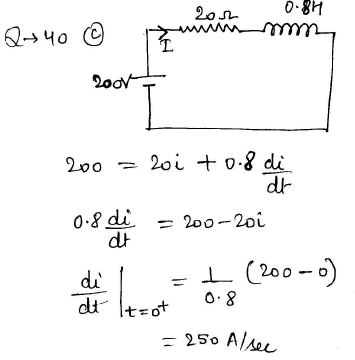Technical Test SSC JE: Electrical Engineering (EE)- 9 - Question 41

What should be the value of resistance R, in the above circuit if it has to absorb the maximum
power from the source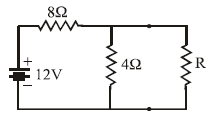Detailed Solution for Technical Test SSC JE: Electrical Engineering (EE)- 9 - Question 41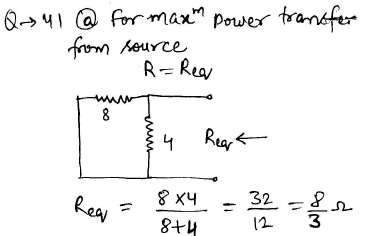Technical Test SSC JE: Electrical Engineering (EE)- 9 - Question 42

The torque on the rotor of a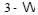motor is more constant than that of a single phase motor because

Technical Test SSC JE: Electrical Engineering (EE)- 9 - Question 43

When the secondary coil of a transformer is short circuited, the primary inductance

Detailed Solution for Technical Test SSC JE: Electrical Engineering (EE)- 9 - Question 43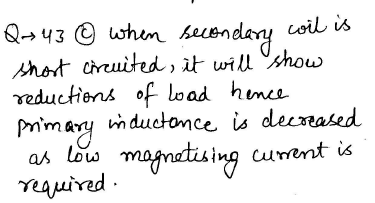Technical Test SSC JE: Electrical Engineering (EE)- 9 - Question 44

Q-factor of a parallel tuned circuit can bei ncreased by

Detailed Solution for Technical Test SSC JE: Electrical Engineering (EE)- 9 - Question 44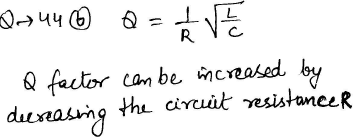Technical Test SSC JE: Electrical Engineering (EE)- 9 - Question 45

Air blast circuit breakers for 400 KV power system are designed to operate in

Technical Test SSC JE: Electrical Engineering (EE)- 9 - Question 46

An overhead line conductor has an inductance per unit length of L Henry. If the entire medium
around the conductor is filled with a dielectric material of permittivity (E), then the inductance
will be

Detailed Solution for Technical Test SSC JE: Electrical Engineering (EE)- 9 - Question 46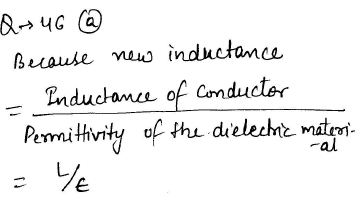Technical Test SSC JE: Electrical Engineering (EE)- 9 - Question 47

In two-wattmeter method of power measurement one of the watt meter will show negative reading when the load pf angle is strictly

Technical Test SSC JE: Electrical Engineering (EE)- 9 - Question 48

A reactance relay is

Technical Test SSC JE: Electrical Engineering (EE)- 9 - Question 49

To limit current chopping in vacuum circuit breakers, the contact

Technical Test SSC JE: Electrical Engineering (EE)- 9 - Question 50

The main difference between speed-time curves of main line service as compared to sub urban services in

Technical Test SSC JE: Electrical Engineering (EE)- 9 - Question 51

Which of the following relay has directional feature

Technical Test SSC JE: Electrical Engineering (EE)- 9 - Question 52

Carbon brushes are preferable to copper brushes because

Technical Test SSC JE: Electrical Engineering (EE)- 9 - Question 53

A power plant has a maximum demand of 15 MW. The load factor is 50% and the plant factor is 40%. The operating reserve is

Detailed Solution for Technical Test SSC JE: Electrical Engineering (EE)- 9 - Question 53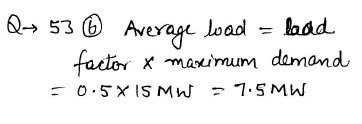Technical Test SSC JE: Electrical Engineering (EE)- 9 - Question 54

The starting torque of a servomotor under unbalanced operation is proportional to

Detailed Solution for Technical Test SSC JE: Electrical Engineering (EE)- 9 - Question 54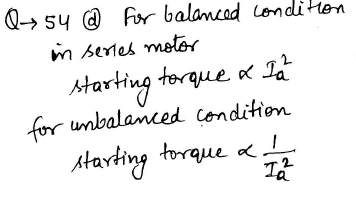Technical Test SSC JE: Electrical Engineering (EE)- 9 - Question 55

Quadrilateral speed-time curve is the closer approximation for

Technical Test SSC JE: Electrical Engineering (EE)- 9 - Question 56

Travelling crane is used for lifting and moving heavy loads. It uses

Technical Test SSC JE: Electrical Engineering (EE)- 9 - Question 57

For Domestic wiring method commonly used is

Technical Test SSC JE: Electrical Engineering (EE)- 9 - Question 58

The time taken for a surge to travel a 600 km long overhead transmission link is

Detailed Solution for Technical Test SSC JE: Electrical Engineering (EE)- 9 - Question 58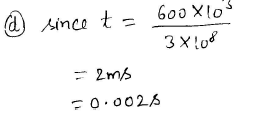Technical Test SSC JE: Electrical Engineering (EE)- 9 - Question 59

Ripple factor of half wave rectifier is

Technical Test SSC JE: Electrical Engineering (EE)- 9 - Question 60

Which one is unipolar transistor

Technical Test SSC JE: Electrical Engineering (EE)- 9 - Question 61

A polarized electrolytic capacitor can be used

Technical Test SSC JE: Electrical Engineering (EE)- 9 - Question 62

162.A moving coil instrument gives full scale deflection with a current of 100 mA when the potential difference across its terminals is 50mV. Calculate the shunt resistance to enable the instrument to read upto 100 A

Detailed Solution for Technical Test SSC JE: Electrical Engineering (EE)- 9 - Question 62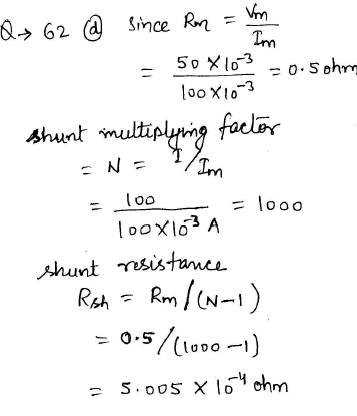Technical Test SSC JE: Electrical Engineering (EE)- 9 - Question 63

Which of the following motors are used in tape recorders

Technical Test SSC JE: Electrical Engineering (EE)- 9 - Question 64

Which motor would you select for vacuum cleaner

Technical Test SSC JE: Electrical Engineering (EE)- 9 - Question 65

A tank circuit consist of

Technical Test SSC JE: Electrical Engineering (EE)- 9 - Question 66

The commutator in a dc machine acts as

Technical Test SSC JE: Electrical Engineering (EE)- 9 - Question 67

Formative time lag depends on the mechanism of the avalanche growth in gap. The formative time lag is usually

Detailed Solution for Technical Test SSC JE: Electrical Engineering (EE)- 9 - Question 67

The formative time lags depend mostly on the mechanism of the avalanche growth in the gap. The formative time lag is usually much shorter than the statistical time lag and therefore the statistical time lag can be determined by measuring the total time lag.

Technical Test SSC JE: Electrical Engineering (EE)- 9 - Question 68

EHV cables are filled with thin oil under pressure

Technical Test SSC JE: Electrical Engineering (EE)- 9 - Question 69

Arcing on transmission lines is prevented by connecting a suitable

Technical Test SSC JE: Electrical Engineering (EE)- 9 - Question 70

In half wave rectifier, if an a.c. supply is 60 Hz, then what is the a.c. ripple at output ?

Technical Test SSC JE: Electrical Engineering (EE)- 9 - Question 71

A power supply has full load voltage of 24V. What is the no load voltage for 5% regulation?

Detailed Solution for Technical Test SSC JE: Electrical Engineering (EE)- 9 - Question 71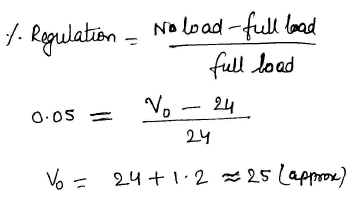Technical Test SSC JE: Electrical Engineering (EE)- 9 - Question 72

For an existing ac transmission line, the string efficiency is 80%. If dc voltage is supplied for the same set up, the string efficiency will be

Technical Test SSC JE: Electrical Engineering (EE)- 9 - Question 73

When excitation is altered in an alternator connected to an infinite line, it provides a corresponding change in

Technical Test SSC JE: Electrical Engineering (EE)- 9 - Question 74

Lightening surges are arrested by

Technical Test SSC JE: Electrical Engineering (EE)- 9 - Question 75

The rms value of a half wave rectified current is 10A. Its value for full wave rectification would be

Technical Test SSC JE: Electrical Engineering (EE)- 9 - Question 76

For the wave given below, the rms value will be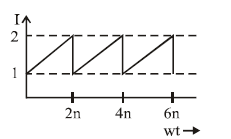Technical Test SSC JE: Electrical Engineering (EE)- 9 - Question 77

The equation for 25 cycles current sine wave having rms value of 30 amperes will be

Technical Test SSC JE: Electrical Engineering (EE)- 9 - Question 78

A 220 V series motor takes a current 35 amp and runs at 500 rpm. Armature resistance =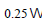and series field resistance =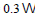. If iron and friction losses amount to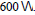Whatis the armature torque

Detailed Solution for Technical Test SSC JE: Electrical Engineering (EE)- 9 - Question 78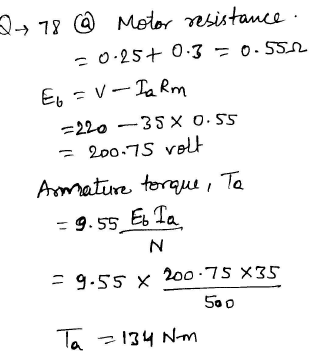Technical Test SSC JE: Electrical Engineering (EE)- 9 - Question 79

Illumination level required for precision work is around

Technical Test SSC JE: Electrical Engineering (EE)- 9 - Question 80

Lumen/watt is the unit of

Technical Test SSC JE: Electrical Engineering (EE)- 9 - Question 81

A DC generator used for ac welding should have

Technical Test SSC JE: Electrical Engineering (EE)- 9 - Question 82

The load taken by welding transformer is

Technical Test SSC JE: Electrical Engineering (EE)- 9 - Question 83

In rectifier circuit shown above, what should be minimum peak-inverse voltage (PIV) rating of the diode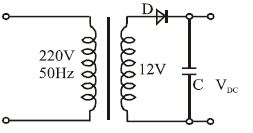Detailed Solution for Technical Test SSC JE: Electrical Engineering (EE)- 9 - Question 83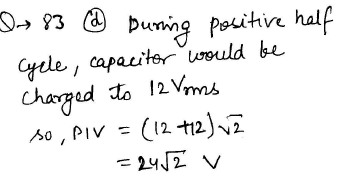Technical Test SSC JE: Electrical Engineering (EE)- 9 - Question 84

In an open circuited p-n junction diode space charge density at the junction is

Technical Test SSC JE: Electrical Engineering (EE)- 9 - Question 85

In an HVDC system

Technical Test SSC JE: Electrical Engineering (EE)- 9 - Question 86

In order to have lower cost of power generation

Technical Test SSC JE: Electrical Engineering (EE)- 9 - Question 87

Mho relay is usually employed for the protection of

Technical Test SSC JE: Electrical Engineering (EE)- 9 - Question 88

The making and breaking currents of 3- f ac circuit breakers in power system are respectively in what form

Technical Test SSC JE: Electrical Engineering (EE)- 9 - Question 89

For a fault in power system, the term critical clearing time is related to

Technical Test SSC JE: Electrical Engineering (EE)- 9 - Question 90

A cable has inductance of 0.22 mH per km and capacitance of 0.22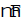per km. The surge
impedance of the cable is

Detailed Solution for Technical Test SSC JE: Electrical Engineering (EE)- 9 - Question 90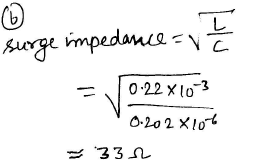Technical Test SSC JE: Electrical Engineering (EE)- 9 - Question 91

The shunt winding of a motor has a resistance of 85W at 22ºC. When the motor runs at full load, its resistance increases to 100 ohms. There sistance temperature coefficient of winding per OºC is 0.004. The rise in temperature of the winding will be nearly

Detailed Solution for Technical Test SSC JE: Electrical Engineering (EE)- 9 - Question 91Technical Test SSC JE: Electrical Engineering (EE)- 9 - Question 92

Temperature coefficient of resistance is expressed in terms of

Technical Test SSC JE: Electrical Engineering (EE)- 9 - Question 93

For the same peak value of voltage which wave will have the highest rms value ?

Technical Test SSC JE: Electrical Engineering (EE)- 9 - Question 94

A wire 2.5m long is bent into a square. A current of 100 A flowing through the wire will producea magnetising force at the centre of the square,equal to

Technical Test SSC JE: Electrical Engineering (EE)- 9 - Question 95

Common base configuration is little used because

Technical Test SSC JE: Electrical Engineering (EE)- 9 - Question 96

The decrease in terminal voltage of a shuntn generator is due to

Technical Test SSC JE: Electrical Engineering (EE)- 9 - Question 97

The armature voltage control is considered as suitable, in case the dc machine is driven at

Technical Test SSC JE: Electrical Engineering (EE)- 9 - Question 98

Interpoles are usually wound with

Technical Test SSC JE: Electrical Engineering (EE)- 9 - Question 99

If the flux of dc motor approaches zero

Technical Test SSC JE: Electrical Engineering (EE)- 9 - Question 100

Which of the following is not a routine test on transformers

## Mock Test Series for SSC JE Electrical Engineering

2 videos|1 docs|55 tests
 Use Code STAYHOME200 and get INR 200 additional OFF Use Coupon Code
Information about Technical Test SSC JE: Electrical Engineering (EE)- 9 Page
In this test you can find the Exam questions for Technical Test SSC JE: Electrical Engineering (EE)- 9 solved & explained in the simplest way possible. Besides giving Questions and answers for Technical Test SSC JE: Electrical Engineering (EE)- 9, EduRev gives you an ample number of Online tests for practice

## Mock Test Series for SSC JE Electrical Engineering

2 videos|1 docs|55 tests